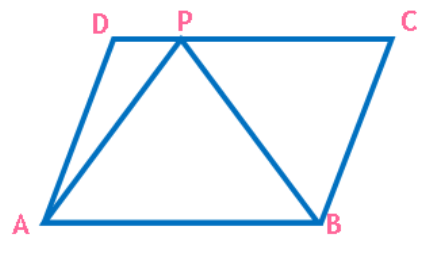Maths / Areas Of Parallelograms And Triangles / Relation between Area of Parallelogram and Triangle

QUESTION

In the figure,ABCD is a parallelogram and ABP is a triangle . If ar (APB)=16 sq. units,ar(ADP)+ar(BCP) will beOPTIONS A. 8 sq. units B. 48 sq.units C. 32 sq. units D. 16 sq.units
Right Option : D

EXPLANATION
Explain TypeExplanation Content
Text

ABCD is a parallelogram and ABP is a triangle on the same base AB and between the same paralles the aea of ABCD = 2 times ABP

ar (APB)=16 sq.

Area of ABCD = 2 X 16 = 32 Sq units.

ar(ADP)+ar(BCP) = ar(ABCD) - ar(APB) = 32 - 16 = 16 sq units

TestimonialsPARENT RESPONSE c/o Prisha Gupta C/o ABHYAS Academy
8th
Being a parent, I saw my daughter improvement in her studies by seeing a good result in all day to day compititive exam TMO, NSO, IEO etc and as well as studies. I have got a fruitful result from my daughter.